STRUCTURALBIOLOGY
ISSN: 2059-7983
Volume 72| Part 9| September 2016| Pages 1073-1075

## From deep TLS validation to ensembles of atomic models built from elemental motions. Addenda and corrigendum

aCentre for Integrative Biology, Institut de Génétique et de Biologie Moléculaire et Cellulaire, 1 rue Laurent Fries, BP 10142, 67404 Illkirch, France, bFaculté des Sciences et Technologies, Université de Lorraine, BP 239, 54506 Vandoeuvre-les-Nancy, France, cMolecular Biophysics and Integrated Bioimaging Division, Lawrence Berkeley National Laboratory, Berkeley, California, USA, dDepartment of Bioengineering and Therapeutic Sciences, University of California, San Francisco, San Francisco, California, USA, and eDepartment of Bioengineering, University of California, Berkeley, Berkeley, California, USA
*Correspondence e-mail: sacha@igbmc.fr

(Received 24 June 2016; accepted 12 August 2016; online 31 August 2016)

Researcher feedback has indicated that in Urzhumtsev et al. [(2015) Acta Cryst. D71, 1668–1683] clarification of key parts of the algorithm for interpretation of TLS matrices in terms of elemental atomic motions and corresponding ensembles of atomic models is required. Also, it has been brought to the attention of the authors that the incorrect PDB code was reported for one of test models. These issues are addressed in this article.

### 1. Incorrect PDB code

In the original article (Urzhumtsev et al., 2015), we used several atomic models in order to test the algorithms and provide examples. Unfortunately, the incorrect PDB code had been reported for one of them. Everywhere in the text (§6.2, Tables 2 and 3), 1rge should be used instead of 1dqv and ribonuclease S should be used instead of synaptotagmin. We apologize for this confusion. The diffraction data set used for test refinement of ribonuclease S was obtained from the CCP4 (Winn et al., 2011) distribution (https://www.ccp4.ac.uk/examples/rnase/rnase25.mtz).

### 2. Origin choice

The problem of the origin choice is discussed in detail in the review of Urzhumtsev et al. (2013) leading us to provide less detail in Urzhumtsev et al. (2015). As mentioned in §2.2 of Urzhumtsev et al. (2015), the T and S matrices depend on the point (origin of the TLS group) with respect to which the three libration axes are defined. This is also important for generating the Un matrices from a set of TLS matrices. Confusion arises from the fact that the TLS origin may be, and in fact usually is, different from the origin of the coordinate system in which the atomic coordinates are provided.

The choice of the TLS origin is arbitrary; some typical choices are described in §2.2. Let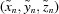, n = 1,…N be atomic Cartesian coordinates as the input parameters of the procedure; for example, they may be the coordinates given in the PDB file. Let (xTLS,yTLS,zTLS ) be respective coordinates of the origin of the TLS group. The origin of basis [M] defined in Urzhumtsev et al. (2015) is assumed to coincide with this point. This means that the coordinates that are input to the ensemble generating procedure (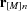in §7.2 of Urzhumtsev et al., 2015) are also expected to be shifted to the origin of the TLS group as follows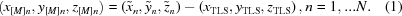The matrix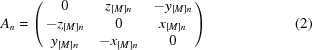[equation (3) in Urzhumtsev et al., 2015] uses these new coordinates (1).

### 3. Un matrices

The TLS model is valid for harmonic motions and, as a consequence, for small libration amplitudes only. It allows for calculation of the individual atomic displacement parameters Un in two different ways. They may be calculated analytically using the formulae (2) and (3) from Urzhumtsev et al. (2015). Alternatively, the same matrices can be calculated numerically from the coordinates of the set of models generated explicitly using the procedure described in §7 and Appendix A of Urzhumtsev et al. (2015). We stress that in formulae (59)–(61) the expressions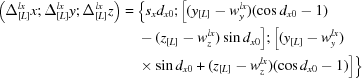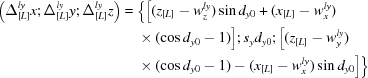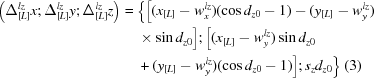are the coordinates of the libration shifts in the basis [L]; similarly, the values (tx0,ty0,tz0 ) in (48) are the coordinates of the vibration shifts in the basis [V]. These coordinates must be converted into the basis [M] in order to obtain the coordinates of the total shifts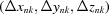, n = 1,…N, to be applied to the atomic coordinates, n = 1, … N. Here k is the number of the generated model.

Once an ensemble is generated, the atomic displacement matrix Un for each atom n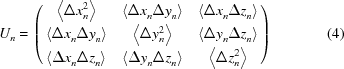can be calculated directly from the coordinates (xnk,ynk,znk ), k = 1,…K of the multiple copies of the same atom in the ensemble [see formula (2.2) from Urzhumtsev et al., 2013]. Here atomic coordinates are in the basis [M] and averaging is performed over all K instances of the atom in the ensemble.

These two somewhat independent routes to obtain the Un matrices allow a convenient way of validating the described procedures. Indeed, given parameters of elemental motions one can construct TLS matrices and then calculate Un from these TLS matrices using the analytical expression cited above. Also, one can use TLS matrices to generate an ensemble of models and then derive Un from the ensemble using formula (4). We added this comparison to cctbx (Grosse-Kunstleve et al., 2002) as a test-exercise of the implementation.

Now the Phenix (Adams et al., 2010) command phenix.tls_as_xyz model.pdb n_models = N creates three PDB files containing the following.

 (i) An ensemble of N models (N can be any positive integer) that are consistent with the TLS model (TLS records must be present in the model.pdb file header). (ii) A single model with anisotropic Un (UTLS in ANISOU records) calculated from the TLS matrices analytically. (iii) A single model with anisotropic Un (Uensemble, ANISOU records) calculated numerically from the ensemble of models.

Un obtained using the two different approaches are expected to be similar with possible differences arising from several sources, such as the following.

 (a) Non-linearity of the TLS approximation (large libration amplitudes; Table 1). (b) A finite number of models in the ensemble; empirically we found that 5000–10 000 is sufficient most of the time (Fig. 1). (c) Numerical errors arising from a long chain of transformations: from decomposing TLS matrices into basic parameters of elemental motions and using these parameters to generate a large set of models that are then used to compute the anisotropic Un matrices.
 Table 1Matrix U calculated analytically for two atoms (see the text for details) from the TLS matrices (UTLS) and numerically from an ensemble of 20 000 models (Uensemble) for different libration amplitude dz
 Matrix elements Uzz, Uxz, Uyz are always equal to 0 and not shown. The last column shows the ratio of the maximal difference between respective UTLS and Uensemble elements compared to the maximal UTLS element. Two lines for dz = 0.10 stand for two independent runs of the random model generation.
dz (rad/°) UTLS (Uxx, Uyy, Uxy) Uensemble (Uxx, Uyy, Uxy) Δmax/Umax
0.05/2.9 0.00250, 0.00063, −0.00125 0.00250, 0.00063, −0.00125 0
0.07/4.0 0.00490, 0.00123, −0.00245 0.00487, 0.00122, −0.00242 0.006
0.09/5.2 0.00810, 0.00202, −0.00405 0.00791, 0.00201, −0.00394 0.023
0.10/5.7 0.01000, 0.00250, −0.00500 0.00993, 0.00254, −0.00496 0.007
0.10/5.7 0.01000, 0.00250, −0.00500 0.00993, 0.00253, −0.00494 0.007
0.15/8.6 0.02250, 0.00562, −0.01125 0.02207, 0.00567, −0.01079 0.020
0.20/11.5 0.04000, 0.01000, −0.02000 0.03802, 0.01025, −0.01861 0.049
0.25/14.3 0.06250, 0.01562, −0.03125 0.05970, 0.01664, −0.02858 0.045
0.30/17.2 0.09000, 0.02250, −0.04500 0.08204, 0.02415, −0.03909 0.088
0.50/28.6 0.25000, 0.06250, −0.12500 0.20432, 0.07408, −0.08651 0.183
0.70/40.1 0.49000, 0.12250, −0.24500 0.32987, 0.15339, −0.11880 0.327
0.90/51.6 0.81000, 0.20250, −0.40500 0.44468, 0.25448, −0.12348 0.451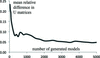Figure 1 Comparison of the matrices UTLS calculated for an atomic model analytically (see the text for details) and the matrices calculated from the ensemble models (Uensemble) as a function of the number of models generated. The mean relative difference between the elements of the two sets of matrices is defined as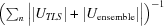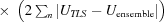where the sums are calculated over all atoms and over all six elements of each pair of U matrices.

Since TLS modeling is based on a linearity approximation (Urzhumtsev et al., 2013) one may expect a significant difference between the matrices Un calculated analytically using TLS matrices and those calculated directly by (4) if the libration amplitudes are large. As mentioned in §6.1 of Urzhumtsev et al. (2015), large values for the vibration and libration amplitudes are not physically meaningful.

Table 1shows matrices U1 = U2 for an artificial example of two atoms with the coordinates (0, 0, 0) and (1, 2, 3) when the only motion applied was libration around the axis parallel to Oz that passes through the center of mass of this system taken as the TLS origin. In the basis [M] the coordinates of these atoms are equal to (−0.5, −1.0, −1.5) and (0.5, 1.0, 1.5), respectively. The matrices were obtained using the two methods discussed above applying different amplitudes dz (for definitions see Urzhumtsev et al., 2015). As discussed previously (§2.3 in Urzhumtsev et al., 2013, and references therein), the discrepancy between two corresponding matrices is significant when the libration amplitude becomes larger than approximately 0.10–0.15 rad (6–9°).

To investigate the number of models sufficient to reproduce UTLS by Uensemble, we took the CA atoms from fragment A6–A61 of protein G IgG-binding domain III model (PDB code 2igd) and fitted TLS matrices to individual anisotropic Un of this model using the phenix.tls tool. Then we calculated UTLS for each atom of the model and independently generated a set of random models from which we calculated Uensemble to compare them with UTLS. Fig. 1shows the mean relative difference between the sets of the U elements as a function of the number of generated models.

### Acknowledgements

We thank Professor D. Case (Rutgers University) for bringing our attention to the incorrectly reported PDB code, and for questions and discussions that prompted the clarifications.

### References

This is an open-access article distributed under the terms of the Creative Commons Attribution (CC-BY) Licence, which permits unrestricted use, distribution, and reproduction in any medium, provided the original authors and source are cited.STRUCTURALBIOLOGY
ISSN: 2059-7983
Volume 72| Part 9| September 2016| Pages 1073-1075